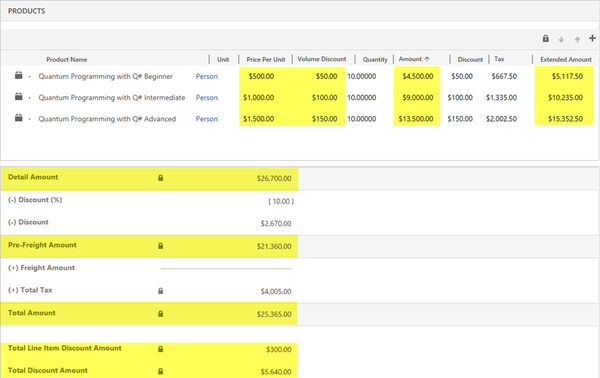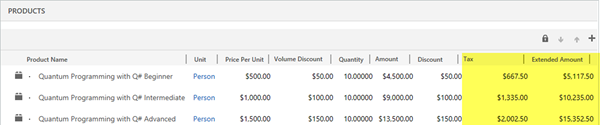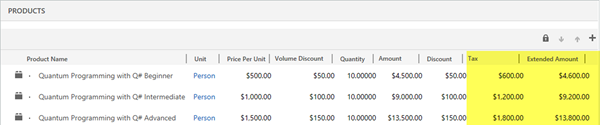# System Pricing Calculations for Quotes and Orders in Dynamics 365

### Colin Maitland, 15 August 2018

In this article I will briefly describe the System Pricing Calculations for Quotes/Orders in Microsoft Dynamics 365.

It is possible to disable the System Pricing Calculations for all the pricing fields on Opportunities, Quotes, Orders and Invoices in Dynamics 365. Refer to https://msdn.microsoft.com/en-us/library/dn817885.aspx.

IMPORTANT NOTE: Disabling this option will require you to then provide custom programming to calculate all the previously system calculated pricing fields for Opportunities, Quotes Orders and Invoices.

This customised subgrid and form for a Quote/Order displayed in the following image highlights the fields that are calculated by Microsoft Dynamics 365; i.e.

• On Quote/Order Products: Price Per Unit, Volume Discount, Amount and Extended Amount.
• On Quotes/Orders: Detail Amount, Pre-Freight Amount, Total Amount, Total Line Item Discount Amount, and Total Discount Amount.On Quote/Order Products the Tax is not system calculated.

By default, the Total Line Item Discount Amount and Total Discount Amount are not displayed on the Quote/Order form.

The pricing fields for Quote/Order Products are calculated as follows:

### PRICE PER UNIT

When the Pricing option is set to ‘Use Default’, the Price Per Unit is calculated based on the configuration of the Price List Item identified using the Price List on the Quote/Order, the Product and the Unit on the Order/Quote Product. The Pricing Method configured on the Price List Item could be one of the following:

• Currency Amount
• Percent of List (i.e. Percent of List Price)
• Percent Markup (of either Current Cost or Standard Cost)
• Percent Margin (of either Current Cost or Standard Cost)

If one of the Percent Pricing Methods is used, then a Rounding Policy may also be configured to determine whether the calculated Price Per Unit is rounded. There are various options for how the Price Per Unit is rounded.

If using the Percent of List Pricing Method, you will need to add the standard List Price field to the Product form so that the List Price can be configured for Products.

When the Pricing option is set to ‘Override Pricing’, as is the case when adding a Write-In Product to the Quote/Order, the Price Per Unit must be manually entered by the User.

### VOLUME DISCOUNT

The Volume Discount is calculated if a Discount List is associated with the Price List Item and the Quantity falls within one of the Quantity Breaks configured in the Discount List.

Discount Lists may be configured to apply the same Discount or different Discounts based on Quantity.

The calculated Discount is a Discount on the Price Per Unit and may be configured as a Percentage or as an Amount. If the Price Per Unit is \$1,000 and the Quantity is 10 and the Discount List associated with the Price List Item provides a 10% Discount or a \$100 Discount for the specified Quantity, then the Volume Discount will be \$100.

### AMOUNT

The Amount is (Price Per Unit - Volume Discount) * Quantity; e.g. (\$1000 - \$100) * 10 = \$9,000.

### EXTENDED AMOUNT

There are two methods by which the Extended Amount might be calculated depending on the ‘Discount Calculation Method’ setting on the Sales tab in System Settings. By default, this setting is configured as ‘Line Item’ and results in the Extended Amount being calculated as follows:

• (((Price Per Unit - Volume Discount) * Quantity) - Discount Amount) + Tax; e.g. ((\$1,000 - \$100) * 10) - \$100) + \$1,335 = \$10,235. Note: In this example, the pre-Tax amount is \$8,990.However, if the ‘Discount Calculation Method’ setting is set to ‘Per Unit’, then the Extended Amount is calculated as follows:

• (((Price Per Unit - Volume Discount) * Quantity) - (Quantity * Discount Amount)) + Tax; e.g. ((\$1,000 - \$100) * 10) - (10 * \$100)) + \$1,200 = \$9,200. Note: In this example, the pre-Tax amount is \$8,000.The difference between the ‘Line Item’ calculation and the ‘Per Unit’ calculation is the addition of the Quantity as a multiplier of the Discount Amount.

In both calculations, the Extended Amount is Tax Inclusive. In this example the Tax is 15% of the calculated pre-Tax amount.

The pricing fields for Quotes/Orders are calculated as follows:

### DETAIL AMOUNT

The Detail Amount is the sum of the ((Amount - Discount) + Tax) from all the related Quote/Order Products; e.g. \$26,700. The Detail Amount is Tax Exclusive, i.e. it is not the sum of the Extended Amount from all the related Quote/Order Products.

### PRE-FREIGHT AMOUNT

The Pre-Freight Amount is (Detail Amount - Discount Percent) - Discount Amount; e.g. (\$26,700 - 10%) - \$2,670 = \$21,360.

It is important to know that the Discount Percent is subtracted prior to the Discount Amount. If this were the case the Pre-Freight Amount calculate to a different value; i.e. in this example \$21,627 instead of \$21,360; a difference of \$267.

### TOTAL TAX

The Total Tax is the sum of the Tax from all the related Quote/Order Products; e.g. \$4,005.

It is important to know that the Total Tax does not take the Discount Percent, Discount Amount, or Freight Amount into consideration. I discuss this issue and provide some replacement calculations in a series of three articles titled ‘Totals and Discounts on Quotes/Order in Dynamics 365’.

### TOTAL AMOUNT

The Total Amount is Pre-Freight Amount + Freight Amount + Total Tax; e.g. \$21,360 + \$0 \$4,005 = \$25,365.

### TOTAL LINE ITEM DISCOUNT AMOUNT

The Total Line Item Discount Amount is the sum of the Manual Discount from all the related Quote/Order Products; e.g. \$300.00

### TOTAL DISCOUNT AMOUNT

The Total Discount Amount is the sum of the Total Line Item Discount Amount + Discount Percent + Discount Amount; e.g. \$2,670 + \$2,670 + \$300 = \$5,640.